# Test: Electrical Machines- 5

## 10 Questions MCQ Test GATE Electrical Engineering (EE) 2023 Mock Test Series | Test: Electrical Machines- 5

Description
Attempt Test: Electrical Machines- 5 | 10 questions in 30 minutes | Mock test for Electrical Engineering (EE) preparation | Free important questions MCQ to study GATE Electrical Engineering (EE) 2023 Mock Test Series for Electrical Engineering (EE) Exam | Download free PDF with solutions
*Answer can only contain numeric values
QUESTION: 1

### A 1000 hp, 4 pole, 3 phase, 440 V, 50 Hz, Induction motor has a speed of 1440 rpm on full load. The number of cycles of the rotor voltage makes per minutes will be______

Solution: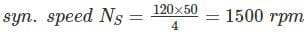Full load speed given as 1440 rpm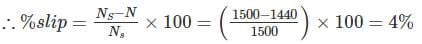Rotor voltage frequency = s × f = 0.04 × 50 = 5%
2 cycles/sec
⇒ 2 × 60 cycle/min = 120 cycles/min

QUESTION: 2

### Under variable frequency operation of 3 phase Induction motor, air gap flux is kept constant by adjusting the motor voltage. For constant air gap flux, machine develops the same torque when the

Solution: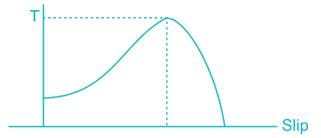In low slip region (stable region)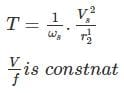Slip speed = SNs = Ns – Nr
Ns ∝ f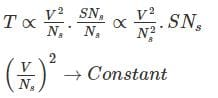Slip speed, SNs → Constant

*Answer can only contain numeric values
QUESTION: 3

### The rotor winding of a 6 pole, 3 phase, slip-ring induction motor receive a 3-phase, 50 Hz supply produced an air gap mmf rotating in the clock wise direction with respect to the rotor the rotor itself is being rotated in the anticlockwise direction at 400 rpm. Frequency of the voltages induced in the stator phases is _______ Hz

Solution: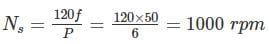Relating speed with respect to rotor = 1000
Speed with respect to stator = Nactual
Speed of rotor = Nr
1000 = Nactual – (-400)
Nactual = 600 R.P.M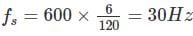QUESTION: 4

A 6 – pole, 3 phase induction motor is drawing current from a 50Hz supply. If the rotor is running at a speed of 970 rpm, what is the speed of rotor magnetic field with respect to rotor?

Solution:

Synchronous speed of stator field, NS = 120f/p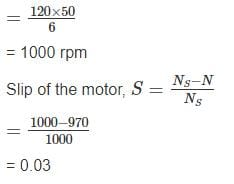Speed of rotor magnetic field relative to rotor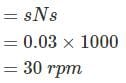*Answer can only contain numeric values
QUESTION: 5

The ratio of starting to full load current for a 15 kW, 300 V and 3ϕ induction motor with DOL starter is _________. For 3 phase inductor motor has full load efficiency 0.90 the full load PF is 0.8 and short-circuit current is 30A at 100V.

Solution:

Ist = 30 A
Starting current when it work at 300V
Ist = 30 × 3 = 90 A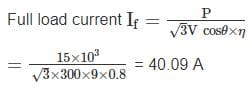∴ Ratio of starting current to full load current
= 90/40.09 = 2.244

QUESTION: 6

A 208 V, 8 pole 60 Hz three phase star connected wound rotor induction motor has a rotor impedance of 0.02 + 0.08 Ω/phase and negligible stator impedance at standstill. Consider the following statements.
1. The breakdown slip at which maximum torque occurs is 0.25
2. The starting torque is 47% of the maximum torque
3. The maximum torque developed by the motor is 1364.4 Nm
Q. Which of the above statements is/are correct?

Solution:

The breakdown slip is,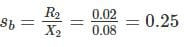The ratio of the torque developed at any slip s to the maximum torque is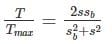For starting torque s = 1, so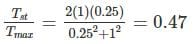Starting torque is 47% of maximum torque.
Synchronous speed,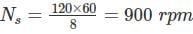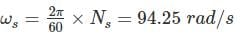Maximum power developed by the motor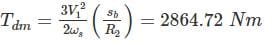*Answer can only contain numeric values
QUESTION: 7

A 2 pole, 50 Hz induction motor supplies 15 kW to a load at a speed of 2950 rotation/min. How much power (In kW) will be supplied by the motor when the torque is doubled.

Solution:

The synchronous speed of this motor is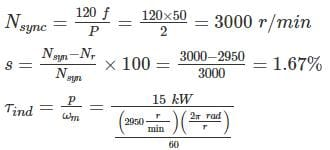= 48.6 N- m
In the low-slip region, the torque-speed curve is linear, and induced torque is directly proportional to slip. Therefore, if torque double, then the new slip will be 3.33 %. The operating speed of the motor is thus
Nr = (1 – .033) Nsyn = (1 -0.033) 3000 = 2900 r/min
∴ Power supplied = τind ω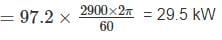*Answer can only contain numeric values
QUESTION: 8

A 50 H.P, 4 pole, 50 Hz 3ϕ SRIM runs at 1440 RPM on full load with rotor current per phase of 30A. If it allows 1000 W for the mechanical loss then find the resistance per phase of the 3ϕ rotor winding.

Solution: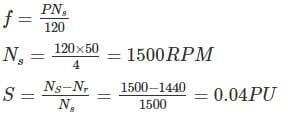We know that rotor copper loss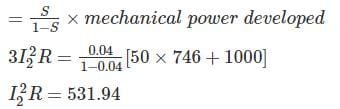900R = 531.94
R = 0.591Ω

QUESTION: 9

A 3 ϕ squirrel cage induction motor has full load slip of 5% and starting current of 5 times the full load current. Neglect the stator and copper losses as well as the rotational losses. then the slip at which maximum torque occurs will be____.  Assume the stator impedance is negligible

Solution: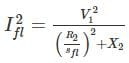Starting current,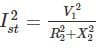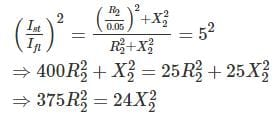R2/X2 = 24/375 = 0.25298

*Answer can only contain numeric values
QUESTION: 10

The rotor resistance and standard reactance of a 3 phase induction motor are respectively 0.015 Ω and 0.09 Ω per phase. At normal voltage the full load slip is 3 %. Find the percentage reduction in stator voltage to develop full load torque at half full load speed.

Solution:

Let Ns = 1000 r.p.m
Full load speed = [1 – 0.03] × 1000 = 970 rpm.
Let the normal voltage be V1 volts.
Speed in second case = 970/2 = 485 r. p.m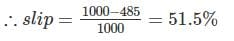We know that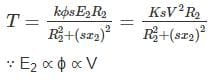Since Torque is the same in both case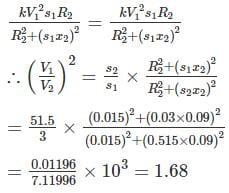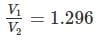Hence % reduction of stator voltage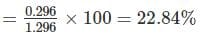Use Code STAYHOME200 and get INR 200 additional OFF Use Coupon Code